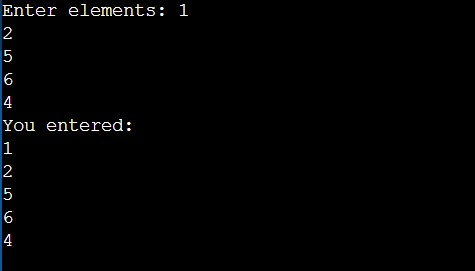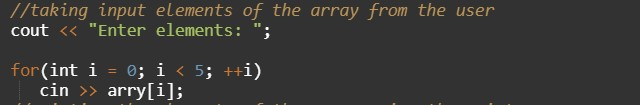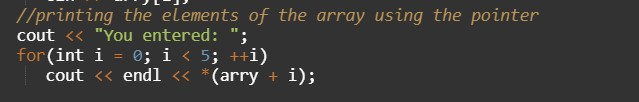C++ Program to Access Elements of an Array Using Pointer

In this tutorial you will learn about the C++ Program to Access Elements of an Array Using Pointer and its application with practical example.

C++ Program to Access Elements of an Array Using Pointer

In this tutorial, we will learn to create a C++ program that will Access Elements of an Array Using Pointer using C++ programming.

Prerequisites

Before starting with this tutorial we assume that you are best aware of the following C++ programming topics:

• Operators in C++ Programming.
• Basic Input and Output function in C++ Programming.
• Basic C++ programming.
• For loop in C++ Programming.
• Using pointer in C++ programming.

Array And Pointer:-

The array in the C++ programming language is a collection of similar data type elements. It can store multiple values in a single variable.

Pointer variables are used to access the element using the address of the variable.

Access Elements of an Array Using Pointer:-

In this program, we will create an array of five elements and take input from the user. Now the printing work of those elements will be done with the help of a pointer variable.

Pointer is a variable that can access elements another variable using its address.

Program:-

Access Elements of an Array Using Pointer.

Output:-In the above program, we have to first initialize the required variable.• arry = it will hold the elements in an array.
• i = it will hold the integer value to control the array.

Taking input from the user in an array the element in the array.Printing the elements of the array using the pointer.In this tutorial we have learn about the C++ Program to Access Elements of an Array Using Pointer and its application with practical example. I hope you will like this tutorial.